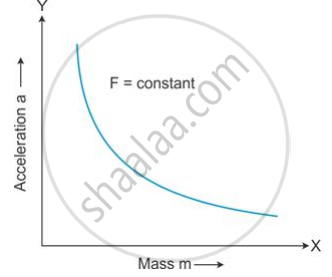# How Does the Acceleration Produced by a Given Force Depend on the Mass of the Body? Draw a Graph to Show It. - Physics

Short Note

How does the acceleration produced by a given force depend on the mass of the body? Draw a graph to show it.

#### Solution

If a given force is applied on bodies of different masses , then the acceleration produced in them is inversely proportional to their masses .
A graph plotted for acceleration (a) against mass (m) is a hyperbola .Concept: Concept of Weight
Is there an error in this question or solution?

#### APPEARS IN

Selina Concise Physics Class 9 ICSE
Chapter 3 Laws of Motion
Exercise 3 (C) | Q 13 | Page 69